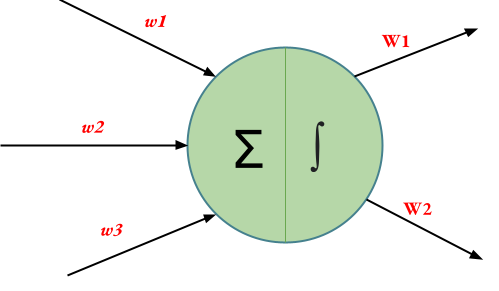# Weight Initialization Techniques for Deep Neural Networks

• Difficulty Level : Expert
• Last Updated : 26 Jan, 2022

While building and training neural networks, it is crucial to initialize the weights appropriately to ensure a model with high accuracy. If the weights are not correctly initialized, it may give rise to the Vanishing Gradient problem or the Exploding Gradient problem. Hence, selecting an appropriate weight initialization strategy is critical when training DL models. In this article, we will learn some of the most common weight initialization techniques, along with their implementation in Python using Keras in TensorFlow.

As pre-requisites, the readers of this article are expected to have a basic knowledge of weights, biases and activation functions. In order to understand what this all, you are and what role they play in Deep Neural Networks – you are advised to read through the article Deep Neural Network With L – Layers

## Terminology or Notations

Following notations must be kept in mind while understanding the Weight Initialization Techniques. These notations may vary at different publications. However, the ones used here are the most common, usually found in research papers.

fan_in = Number of input paths towards the neuron

fan_out = Number of output paths towards the neuron

Example: Consider the following neuron as a part of a Deep Neural Network.For the above neuron,

fan_in = 3 (Number of input paths towards the neuron)

fan_out = 2 (Number of output paths towards the neuron)

## Weight Initialization Techniques

### 1. Zero Initialization

As the name suggests, all the weights are assigned zero as the initial value is zero initialization. This kind of initialization is highly ineffective as neurons learn the same feature during each iteration. Rather, during any kind of constant initialization, the same issue happens to occur. Thus, constant initializations are not preferred.

Zero initialization can be implemented in Keras layers in Python as follows:

## Python3

 # Zero Initializationfrom tensorflow.keras import layersfrom tensorflow.keras import initializers  initializer = tf.keras.initializers.Zeros()layer = tf.keras.layers.Dense(  3, kernel_initializer=initializer)

### 2. Random Initialization

In an attempt to overcome the shortcomings of Zero or Constant Initialization, random initialization assigns random values except for zeros as weights to neuron paths. However, assigning values randomly to the weights, problems such as Overfitting, Vanishing Gradient Problem, Exploding Gradient Problem might occur.

Random Initialization can be of two kinds:

• Random Normal
• Random Uniform

a) Random Normal: The weights are initialized from values in a normal distribution.Random Normal initialization can be implemented in Keras layers in Python as follows:

## Python3

 # Random Normal Distributionfrom tensorflow.keras import layersfrom tensorflow.keras import initializers  initializer = tf.keras.initializers.RandomNormal(  mean=0., stddev=1.)layer = tf.keras.layers.Dense(3, kernel_initializer=initializer)

b) Random Uniform: The weights are initialized from values in a uniform distribution.Random Uniform initialization can be implemented in Keras layers in Python as follows:

## Python3

 # Random Uniform Initializationfrom tensorflow.keras import layersfrom tensorflow.keras import initializers  initializer = tf.keras.initializers.RandomUniform(  minval=0.,maxval=1.)layer = tf.keras.layers.Dense(3, kernel_initializer=initializer)

### 3. Xavier/Glorot Initialization

In Xavier/Glorot weight initialization, the weights are assigned from values of a uniform distribution as follows:Xavier/Glorot Initialization often termed as Xavier Uniform Initialization, is suitable for layers where the activation function used is Sigmoid. Xavier/Gorat initialization can be implemented in Keras layers in Python as follows:

## Python3

 # Xavier/Glorot Unifrom Initializationfrom tensorflow.keras import layersfrom tensorflow.keras import initializers  initializer = tf.keras.initializers.GlorotUniform()layer = tf.keras.layers.Dense(3, kernel_initializer=initializer)

### 4. Normalized Xavier/Glorot Initialization

In Normalized Xavier/Glorot weight initialization, the weights are assigned from values of a normal distribution as follows:Here,is given by:Xavier/Glorot Initialization, too, is suitable for layers where the activation function used is Sigmoid. Normalized Xavier/Gorat initialization can be implemented in Keras layers in Python as follows:

## Python3

 # Normailzed Xavier/Glorot Unifrom Initializationfrom tensorflow.keras import layersfrom tensorflow.keras import initializers  initializer = tf.keras.initializers.GlorotNormal()layer = tf.keras.layers.Dense(3, kernel_initializer=initializer)

### 5. He Uniform Initialization

In He Uniform weight initialization, the weights are assigned from values of a uniform distribution as follows:He Uniform Initialization is suitable for layers where ReLU activation function is used. He Uniform Initialization can be implemented in Keras layers in Python as follows:

## Python3

 # He Uniform Initializationfrom tensorflow.keras import layersfrom tensorflow.keras import initializers  initializer = tf.keras.initializers.HeUniform()layer = tf.keras.layers.Dense(3, kernel_initializer=initializer)

### 6. He Normal Initialization

In He Normal weight initialization, the weights are assigned from values of a normal distribution as follows:Here, \sigma is given by:He Uniform Initialization, too, is suitable for layers where ReLU activation function is used. He Uniform Initialization can be implemented in Keras layers in Python as follows:

## Python3

 # He Normal Initializationfrom tensorflow.keras import layersfrom tensorflow.keras import initializers  initializer = tf.keras.initializers.HeNormal()layer = tf.keras.layers.Dense(3, kernel_initializer=initializer)

### Conclusion:

Weight Initialization is a very imperative concept in Deep Neural Networks and using the right Initialization technique can heavily affect the accuracy of the Deep Learning Model. Thus, an appropriate weight initialization technique must be employed, taking various factors such as activation function used, into consideration.

My Personal Notes arrow_drop_up